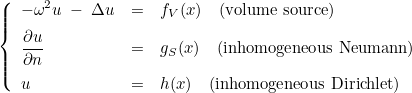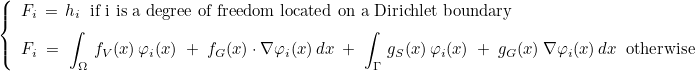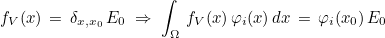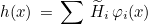# Computation of right hand sides in Montjoie

The following source is implemented in Montjoie (in the case of Helmholtz equation) :For other equations, the implemented sources are similar. More precisely, the implemented source is equal to the following expression :where fG and gG are functions that you can set to 0. The source fV may be set equal to a Dirac :where E0 is called polarization. This polarization can also be used in other expressions of fV. It is also possible to compute the projection of a function h in the finite element basis (by calling AddVolumetricProjection) :In order to declare a new source, the following example can be used

```template<class TypeElement, class TypeSource, class TypeEquation>
class MySource
: public NullSource_Elliptic <TypeElement, TypeSource, TypeEquation>
{
public :
// constructor with a given problem
MySource(const EllipticProblem<TypeElement, TypeEquation>& var)
: NullSource_Elliptic <TypeElement, TypeEquation>(var)
{
// you can initialize the source
}

// true if f_V is non null
bool IsNonNullVolumetricSource(const VectR_N& s) { return true; }

// true if f_G is non null
bool IsNonNullGradientSource(const VectR_N& s) { return true; }

// true if g_S is non null
bool IsNonNullSurfacicSource(int ref) { return true; }

// true if g_G is non null
bool IsNonNullSurfacicSourceGradient(int ref) { return true; }

// value of h (for Dirichlet condition or projection of a function h)
template<class Vector1>
void EvaluateFunction(int i, int j, const R_N& x, Vector1& h)
{
// h is a vector, its size being equal to the number of unknowns
// of the equation
h(0) = 2.0*x(0);
h(1) = -1.5*x(1) + 4.0;
}

// value of f_V
template<class Vector1>
void EvaluateVolumetricSource(int i, int j, const R_N& x, Vector1& fV)
{
fV.Fill(0);
}

// value of f_G
template<class Vector1>
void EvaluateGradientSource(int i, int j, const R_N& x, Vector1& fG)
{
fG.Fill(0);
}

// value of g_S
template<class Vector1>
void EvaluateSurfacicSource(int k, const SetPoints<Dimension>& PointsElem,
const SetMatrices<Dimension>& MatricesElem,
Vector1& gS)
{
gS.Fill(0);
}

// value of g_G
template<class Vector1>
void EvaluateSurfacicSourceGradient(int k, const SetPoints<Dimension>& PointsElem,
const SetMatrices<Dimension>& MatricesElem,
Vector1& gG)
{
gG.Fill(0);
}

bool IsDiracSource()
{ // true if a Dirac source is added
return false;
}

};

// construction of EllipticProblem object
EllipticProblem<TypeElement, TypeEquation>var;
// ...

// then you call the computation of the source
MySource<TypeElement, TypeSource, TypeEquation> source;
// a vector of vectors is provided for the case of computations
// on cyclic domains (or periodic), since a different source may be
// computed for each mode
Vector<Vector<Complexe> > F(1);
var.ComputeGenericSource(F, source);

```

The class TypeSource may be used (or not) as a parameter class where you define only a function. For example, the class VolumetricSource is available if you have only fV non-null (other sources are equal to 0). In that case, it suffices to write a simple class if you wish to specify the function fV

```class MyParamSource
{
public :
// evaluation of fV
template<class R_N, class Vector1>
void EvaluateFunction(int i, int j, const R_N& x, Vector1& f)
{
f(0) = 0.5*sin(pi_wp*x(0));
}
};

// construction of EllipticProblem object
EllipticProblem<TypeElement, TypeEquation>var;
// ...

// then you call the computation of the source
VolumetricSource<TypeElement, MyParamSource, TypeEquation> source;
// a vector of vectors is provided for the case of computations
// on cyclic domains (or periodic), since a different source may be
// computed for each mode
Vector<Vector<Complexe> > F(1);
var.ComputeGenericSource(F, source);

```

The following type of sources are available in Montjoie

The last class is located in files UserSource.hxx/UserSource.cxx and can be modified by anyone to fit its needs.

## Methods of class VarSourceProblem (a base class of EllipticProblem)

 ComputeRightHandSide computation of the source (right hand side of the equation) OnlyOneSource returns true if there is only one source to compute GetNbRhs returns the number of right hand sides AddVolumetricSource adds to a vector the integral of a function against basis functions ModifyRhsStaticCondensation modification of right hand sides due to static condensation (if used) RecomposeSolution recovery of the solution if static condensation is used SetDirichletSource Sets values of u on degrees of freedom located on Dirichlet boundaries SetDirichletSource adds projection of a function on degrees of freedom to a vector SetDirichletSource adds projection of a function on degrees of freedom (of a subset of boundaries) to a vector AddSurfacicSource adds to a vector the surfacic integral of a function against basis functions AddDiracSource adds to a vector the values of basis functions at a given point (equivalent to a Dirac source) ComputeGenericSource computation of a generic source by providing a class defining fV, fG, gS, gG, etc AddIncidentWave adds projection of incident field on degrees of freedom to a vector

## Methods of class NullSource_Elliptic, VolumetricSource, etc

 GetOrigin returns center of the source GetPolarization returns polarization of the source SetPolarization sets polarization of the source InitElement initialization of some variables for computation of source on an element of the mesh EvaluateFunction evaluation of the function used for projection on Dirichlet dofs EvaluateVolumetricSource evaluation of the function fV IsNonNullVolumetricSource returns true if the function fV is non-null EvaluateGradientSource evaluation of the function fG IsNonNullGradientSource returns true if the function fG is non-null InitSurface initialization of some variables for computation of source on a face of the mesh EvaluateSurfacicSource evaluation of the function gV IsNonNullSurfacicSource returns true if the function gV is non-null EvaluateSurfacicSourceGradient evaluation of the function gG IsNonNullSurfacicSourceGradient returns true if the function gG is non-null IsNonNullDirichletSource returns true if inhomogeneous Dirichlet condition is set IsDiracSource returns true if a Dirac function is used for fV ModifyPoints points are translated or rotated (for cyclic/periodic domains) ModifyEvaluationVolume modification of evaluations of fV and fG for cyclic/periodic domains ModifyEvaluationSurface modification of evaluations of gV and gG for cyclic/periodic domains ModifyEvaluationProjection modification of evaluations of h for cyclic/periodic domains

## Public attributes and methods of class TimeSourceHyperbolic

 frequency frequency associated with the time source fsrc class containing the definition of the time source Init initialisation of the time source GenerateValues computation of the time source on interpolation points EvaluateDerivative evaluation of n-th derivative of the time source

## Public attributes and methods of class VarUnsteadSource_Base

 param_initial_condition parameters for the initial condition var_harmonic object for the computation of finite element matrix tinit_source initial time of the source tlimit_source final time of the source tdelta_source characteristic time of the source ComputeRightHandSide computation of the right hand side FillSource sets a vector to be equal to the right hand side InitSource initialisation for time sources AddPrimitiveSourceAtTime adds n-th derivative in time of the right hand side AddScalarSourceAtTime adds n-th derivative in time of the scalar right hand side AddVectorialSourceAtTime adds n-th derivative in time of the vectorial right hand side SetDirichletCondition sets inhomogeneous Dirichlet condition GetFinalTimeSource returns final time of the source